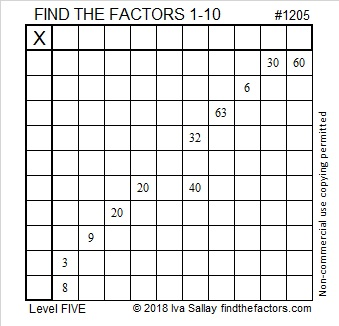# 1205 and Level 5

These Level 5 puzzles always have at least one set of clues whose common factor can only be one number. Find it, and you’ll be able to proceed using just logic and basic multiplication facts. Have fun with it!Print the puzzles or type the solution in this excel file: 10-factors-1199-1210

Here are some facts about the number 1205:

• 1205 is a composite number.
• Prime factorization: 1205 = 5 × 241
• The exponents in the prime factorization are 1 and 1. Adding one to each and multiplying we get (1 + 1)(1 + 1) = 2 × 2 = 4. Therefore 1205 has exactly 4 factors.
• Factors of 1205: 1, 5, 241, 1205
• Factor pairs: 1205 = 1 × 1205 or 5 × 241
• 1205 has no square factors that allow its square root to be simplified. √1205 ≈ 34.713126² + 23² = 1205
34² +  7² = 1205

1205 is the hypotenuse of FOUR Pythagorean triples:
147-1196-1205 calculated from 26² – 23², 2(26)(23), 26² + 23²
476-1107-1205 calculated from 2(34)(7), 34² –  7², 34² +  7²
600-1045-1205 which is 5 times (120-209-241)
723-964-1205 which is (3-4-5) times 241

This site uses Akismet to reduce spam. Learn how your comment data is processed.